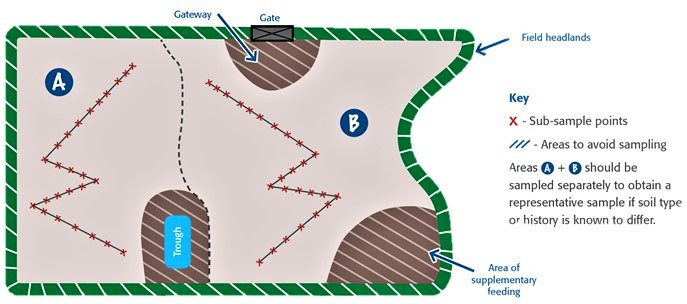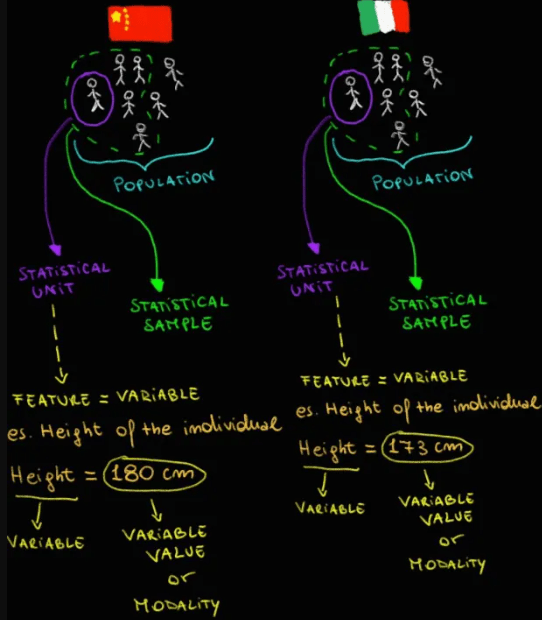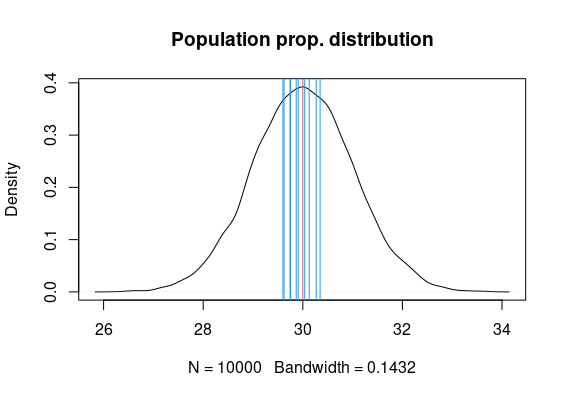Finally, we've reached the end of my personal summary on statistics. As mentioned in one of the early articles in this series on statistics, I view this discipline as divided into three main branches: descriptive statistics, probability theory, and inferential statistics. We've dedicated two articles to descriptive statistics, clarifying how it is useful for describing the characteristics of a phenomenon and, consequently, a variable under examination. We've done the same for probability theory, affirming and demonstrating how it can help us quantify the likelihood of a certain event occurring. Now, we'll do the same with inferential statistics, which, as the name suggests, allows us to infer (deduce, derive) the characteristics of a variable at the population level based on the study of these characteristics at the level of one or more samples from the population. In short, as I've heard many times, inferential statistics allows us to move from the sample to the population.

But a question naturally arises: why is it necessary to study a variable at the sample level and then infer the observations made on the population? Can't we study the variable directly at the population level?

In statistics, it's often necessary to analyze phenomena involving a large number of individuals or, more generally, statistical units. This entails significant economic and technical limitations, to the extent that sometimes it's even impossible to study a phenomenon at the level of an entire population. Consequently, inferential statistics is not only recommended but necessary. Let's consider a practical example. Imagine you want to conduct a satisfaction survey regarding a certain government decision among the Italian population. Would you agree with me that interviewing the entire Italian population is too costly, as well as technically challenging if not impossible? The only option is to select one or more subgroups of the Italian population, interview individuals from these groups, and then extrapolate the results obtained to the entire population.

A statistical investigation that requires the application of inferential statistics is very similar to a criminal case investigation conducted by a detective. The detective uses tools such as a magnifying glass, a notebook, tweezers to gather evidence, and much more to study the crime scene and, from that study, hypothesize the motive and perpetrator of the crime. Similarly, inferential statistics utilizes tools like mathematics, descriptive statistics, and probability theory to study the characteristics of a variable at the sample level and then infer them at the level of the population of interest.

In general, an inferential statistics study follows four consecutive steps:

1. Sampling from the population.

2. Calculating sample statistics, i.e., summary values related to the observed variable at the sample level.

3. Estimating the characteristics of the variable at the population level based on the observations made on the sample.

Just to clarify:

A sample is a subset of a population of statistical units. It must be representative of the population under examination. For example, if we want to study the average height of Italian adults, it doesn't make much sense to include samples of adolescents or children, as they are still in the midst of development and could skew the average. To ensure representativeness of the samples, it's necessary to select them randomly, without being influenced by subjective judgments. For instance, in agronomic studies, plants or regions of agricultural soil are often selected from a given population by randomly tossing a metal circle and following a path marked as X, Y, or W. The plants that randomly fall inside the circle become the statistical units that make up the sample.Look at this summary diagram to review the concepts of statistical unit, sample and population:So, let's clarify this concept:

The primary goal of inferential statistics is to estimate population parameters, such as the mean and standard deviation, based on observations from one or more randomly drawn samples from that population.

To perform this estimation, estimators are required—functions that take data from the samples as input and produce as output an estimate of the desired parameter.

To understand how the estimation of a population parameter, such as the mean, is accomplished based on samples drawn from the population, it's necessary to introduce the secret weapon of inferential statistics, the one that practically allows us to move from samples to the population. We're talking about the Central Limit Theorem, which states:

If the number of samples is sufficiently large (>30 statistical units) and the probabilities of the values of the variable under examination are identically distributed across the samples, then the probability distribution of the means of each sample becomes normal, regardless of the probability distribution of the population, which is unknown.

This theorem is a real ace up the sleeve, as it demonstrates how the mean of the means of each sample converges to the population mean as the number of samples and sample size (number of statistical units in the sample) increase. In such a case, we can say that the function calculating the arithmetic mean of the means of each sample is the estimator, and its value is the estimate of the population mean.

At this point, the most skeptical and attentive among you may be wondering how it's possible to estimate the characteristics of the variable (e.g., mean, variability, etc.) at the population level based on observations made on the sample, if we don't know the actual values of these characteristics at the population level. How can we judge the accuracy of the estimates made? For example, how do we know if the estimate of the average height of the Italian population is correct if we don't know the real population mean value? In practice, it's like asking a sniper to hit a target with their eyes closed without knowing where the target is.This is indeed a challenge, but once again, studies conducted in the past by statisticians greatly simplify the situation. In short, in the past statisticians have conducted various studies related to several types of variables on all possible samples drawn from entire populations. From the analysis of the parameters of the variables considered at the sample and population levels, they have identified general rules that now allow us to estimate the characteristics of variables at the population level based on observations made on one or a few samples, without having to extract and analyze all the population samples each time.

What is the average tomato berry yield on Mr. Rossi's farm?

We know that the population under examination consists of 10,000 tomato plants. Furthermore, it's important to note that in the example below, we assume knowledge of the probability distribution of the population as well as the mean and standard deviation. However, remember that we usually don't have this information. In fact, the population mean is generally unknown and is the parameter we want to estimate.

``````set.seed(1) # for reproducibility

# What we know:

# Population size
population_size <- 10000 # tomato plants
######################################################################################################################
# IMPORTANT!!!!!!
#
# We assume that we know the probability distribution of the population, as well as the mean and standard deviation.
# But remember, this is just to give an example, usually we don't have this information about
# the population and also the average of this is the parameter we want to estimate.
# But this is an example to make you understand better.
#
######################################################################################################################
# Generate a random normal distribution with 10000 values
true_mean_pop <- 30
true_sd_pop <- 1
population_prob_distribution <- rnorm(n = population_size, mean = true_mean_pop, sd = true_sd_pop) # normal distr.
# Visualize population probability distribution
plot(density(population_prob_distribution), main = "Population prop. distribution")
abline(v=true_mean_pop, col=2)````````````# Samples info
set.seed(2)
N <- 10 # number of samples
n <- 10 # sample size
# Extract samples randomly from the population
mean_samples <- c(NA)
dev.std_samples <- c(NA)

for (i in 1:N){
sample <- sample(population_prob_distribution, n)
mean_samples[i] <- mean(sample)
dev.std_samples[i] <- sd(sample)
abline(v=mean(sample), col=4)
}``````

Consider that we have taken 10 samples from the population, each with a size of 10 statistical units. Each blue vertical line in the graph below represents the arithmetic mean calculated for the samples (indeed, we have 10 blue lines).``````# Plot probability distribution
# Considering means of samples
print(mean_samples)
means_samples_prob_distribution <- mean_samples
plot(density(means_samples_prob_distribution), main = "Means samples prob distribution")
population_mean_estimation <- mean(means_samples_prob_distribution)
print(population_mean_estimation)
abline(v=population_mean_estimation, col=2)``````

Here's the Central Limit Theorem in action.

Remember:

The probability distribution of the means of each sample becomes normal, regardless of the probability distribution of the population, which is unknown. Moreover, the mean of the means of each sample converges to the population mean as the number of samples and sample size increase.``````# Considering standard deviations of samples
print(dev.std_samples)
sd_samples_prob_distribution <- dev.std_samples
plot(density(sd_samples_prob_distribution), main = "sd samples prob distribution")
population_sd_estimation <- mean(sd_samples_prob_distribution)
print(population_sd_estimation)
abline(v=population_sd_estimation, col=2)``````

We can also estimate the standard deviation using the estimator, which is the average of the standard deviations of the individual samples.``````# Compare the true population mean and the estimate of this mean.
# Do the same for the standard deviation.
print(paste(round(population_mean_estimation, digits = 4), "vs", true_mean_pop))
print(paste(round(population_sd_estimation, digits = 4), "vs", true_sd_pop))``````

So, we've just seen how we can use the mean estimator to obtain an estimate of the population mean and an estimate of the population standard deviation. In particular, we obtained an estimate of the population mean of 29.928 (remember that in this case, we know that the true population mean is 30) and an estimate of the population standard deviation of 1.0062 (remember that in this case, we know that the true population standard deviation is 1).

I understand. You may be wondering how we can be sure of the accuracy of the estimate. There's an answer for this too. To measure the correctness of the estimate based on sample values, you can use the standard error.The standard error informs us about how precise the estimator used is. In fact, the smaller the standard error, the greater the precision of the estimator.

``````# Calculate standard error
std.err <- sd(means_samples_prob_distribution)/sqrt(n)``````

In our example, the standard error is 0.08243854. Therefore, we can state that the estimator used (mean of sample means) is very precise. Furthermore, from the standard error formula, you can infer that as the sample size (n) increases, the standard error decreases, making the estimator more precise. Conversely, as the standard deviation of the sample mean or the population increases, the standard error increases, reducing the precision of the estimator.

Not convinced? Try changing the parameters N or n and observe how the value of the standard error changes in the example above.

It's crucial not to confuse the standard error with the standard deviation of an estimator. To understand this difference, I recommend watching Joshua Starmer's video.

Precision isn't the only intrinsic characteristic of an estimator; you must also consider other properties of estimators, such as:

• Correctness or unbiasedness of the estimator: This indicates whether an estimator is capable of providing an estimate equal to the true value.• Efficiency of the estimator: Two different estimators that estimate the same unknown parameter can be compared in terms of efficiency in estimating that parameter. The efficiency of an estimator is expressed through the Mean Squared Error, which is calculated as follows:The estimator with the lowest Mean Squared Error is considered the most efficient.

• Consistency of the estimator: This indicates how often the estimator tends to estimate the true value of the considered parameter. In practice, thanks to the Central Limit Theorem, we know that as n increases (or more realistically, with a larger sample), the estimator tends to produce an estimate closer and closer to the true value with a variance that tends to zero.

Well, for now, we'll stop here. I hope this article has been helpful, and as always, please feel free to leave comments and corrections if necessary. See you in the next article, where we'll continue to discuss inferential statistics.

Goodbye, and see you soon.

Omar Almolla